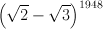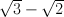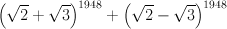The trick to solving this riddle is to switch the plus for a minus and to consider the expression.
Becauseis clearly less than a third, one can be sure, without difficult calculations, thatis a very small number, having hundreds of leading zeros after the decimal point.
On the other hand,is easily found to be a whole number: the even positions in the binomial expansion of both sides are whole numbers, while the odd positions cancel each other.
The value ofis therefore only a very small number shy of a whole number. Its 48th digit after the decimal point is therefore '9', as are all other of the first 900 digits and more after the decimal point.